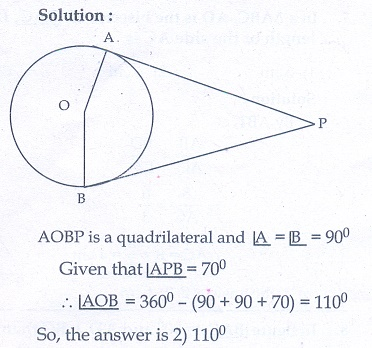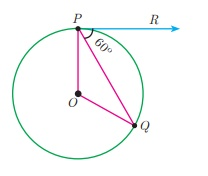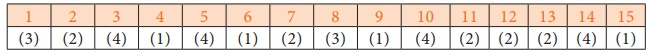Home | | Maths 10th Std | Multiple choice questions

# Multiple choice questions

Mathematics : Geometry: Multiple choice questions with answers / choose the correct answer with answers - Maths Book back 1 mark questions and answers with solution for Exercise Problems

Multiple choice questions

1. If in triangles ABC and EDFthen they will be similar, when

(1) B = E

(2) A = D

(3) B = D

(4) A = F2. In ΔLMN= 60°,  = 50° . If ΔLMN ~ ΔPQR then the value of is

(1) 40°

(2) 70°

(3) 30°

(4) 110°3. If ΔABC is an isosceles triangle with C = 90° and AC = 5 cm, then AB is

(1) 2.5 cm

(2) 5 cm

(3) 10 cm

(4) 5√2 cm4. In a given figure ST || QRPS = 2 cm and SQ = 3 cm. Then the ratio of the area of ΔPQR to the area of ΔPST is(1) 25: 4

(2) 25: 7

(3) 25: 11

(4) 25: 135. The perimeters of two similar triangles ΔABC and ΔPQR are 36 cm and 24 cm respectively. If PQ = 10 cm, then the length of AB is

(1) 6(2/3) cm

(2) 10√6 /3 cm

(3) 66(2/3) cm

(4) 15 cm6. If in ΔABC , DE || BC . AB = 3.6 cm, AC = 2.4 cm and AD = 2.1 cm then the length of AE is

(1) 1.4 cm

(2) 1.8 cm

(3) 1.2 cm

(4) 1.05 cm7. In a ΔABC , AD is the bisector of BAC . If AB = 8 cm, BD = 6 cm and DC = 3 cm. The length of the side AC is

(1) 6 cm

(2) 4 cm

(3) 3 cm

(4) 8 cm8. In the adjacent figure BAC = 90° and AD ┴ BC then(1) BD  CD = BC 2

(2) AB .AC = BC 29. Two poles of heights 6 m and 11 m stand vertically on a plane ground. If the distance between their feet is 12 m, what is the distance between their tops?

(1) 13 m

(2) 14 m

(3) 15 m

(4) 12.8 m10. In the given  figure,  PR = 26 cm,  QR = 24  cm. PAQ = 90° , PA=6 cm and QA = 8 cm. Find PQR(1) 80°

(2) 85°

(3) 75°

(4) 90°11. A tangent is perpendicular to the radius at the

(1) Centre

(2) point of contact

(3) infinity

(4) chord

12. How many tangents can be drawn to the circle from an exterior point?

(1) One

(2) Two

(3) Infinite

(4) Zero

13. The two tangents from an external points P to a circle with centre at O are PA and PB. If APB = 70° then the value of AOB is

(1) 100°

(2) 110°

(3) 120°

(4) 130°14. In figure CP and CQ are tangents to a circle with centre at OARB is another tangent touching the circle at R. If CP = 11 cm and BC = 7 cm, then the length of BR is(1) 6 cm

(2) 5 cm

(3) 8 cm

(4) 4 cm15. In figure if PR is tangent to the circle at P and O is the centre of the circle, then POQ is(1) 120°

(2) 100°

(3) 110°

(4) 90°Tags : Geometry | Mathematics , 10th Mathematics : UNIT 4 : Geometry
Study Material, Lecturing Notes, Assignment, Reference, Wiki description explanation, brief detail
10th Mathematics : UNIT 4 : Geometry : Multiple choice questions | Geometry | Mathematics